# Digital Counters

Counter is a sequential circuit. A digital circuit which is used for a counting pulses is known counter. Counter is the widest application of flip-flops. It is a group of flip-flops with a clock signal applied. Counters are of two types.

• Asynchronous or ripple counters.
• Synchronous counters.

## Asynchronous or ripple counters

The logic diagram of a 2-bit ripple up counter is shown in figure. The toggle (T) flip-flop are being used. But we can use the JK flip-flop also with J and K connected permanently to logic 1. External clock is applied to the clock input of flip-flop A and QA output is applied to the clock input of the next flip-flop i.e. FF-B.

### Logical Diagram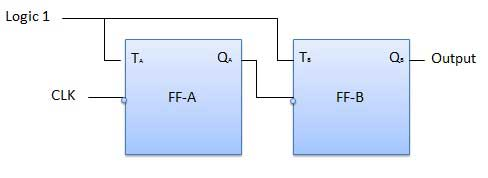### Operation

S.N. Condition Operation
1 Initially let both the FFs be in the reset state QBQA = 00 initially
2 After 1st negative clock edge

As soon as the first negative clock edge is applied, FF-A will toggle and QA will be equal to 1.

QA is connected to clock input of FF-B. Since QA has changed from 0 to 1, it is treated as the positive clock edge by FF-B. There is no change in QB because FF-B is a negative edge triggered FF.

QBQA = 01 after the first clock pulse.

3 After 2nd negative clock edge

On the arrival of second negative clock edge, FF-A toggles again and QA = 0.

The change in QA acts as a negative clock edge for FF-B. So it will also toggle, and QB will be 1.

QBQA = 10 after the second clock pulse.

4 After 3rd negative clock edge

On the arrival of 3rd negative clock edge, FF-A toggles again and QA become 1 from 0.

Since this is a positive going change, FF-B does not respond to it and remains inactive. So QB does not change and continues to be equal to 1.

QBQA = 11 after the third clock pulse.

5 After 4th negative clock edge

On the arrival of 4th negative clock edge, FF-A toggles again and QA becomes 1 from 0.

This negative change in QA acts as clock pulse for FF-B. Hence it toggles to change QB from 1 to 0.

QBQA = 00 after the fourth clock pulse.

### Truth Table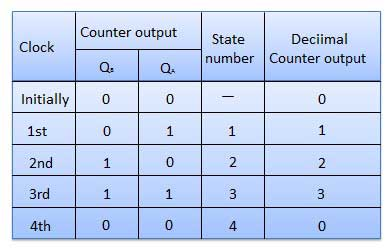## Synchronous counters

If the "clock" pulses are applied to all the flip-flops in a counter simultaneously, then such a counter is called as synchronous counter.

### 2-bit Synchronous up counter

The JA and KA inputs of FF-A are tied to logic 1. So FF-A will work as a toggle flip-flop. The JB and KB inputs are connected to QA.

### Logical Diagram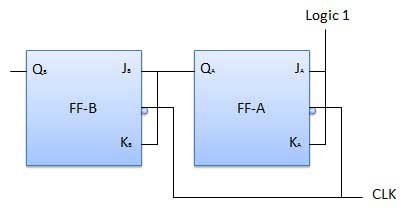### Operation

S.N. Condition Operation
1 Initially let both the FFs be in the reset state QBQA = 00 initially.
2 After 1st negative clock edge

As soon as the first negative clock edge is applied, FF-A will toggle and QA will change from 0 to 1.

But at the instant of application of negative clock edge, QA , JB = KB = 0. Hence FF-B will not change its state. So QB will remain 0.

QBQA = 01 after the first clock pulse.

3 After 2nd negative clock edge

On the arrival of second negative clock edge, FF-A toggles again and QA changes from 1 to 0.

But at this instant QA was 1. So JB = KB= 1 and FF-B will toggle. Hence QB changes from 0 to 1.

QBQA = 10 after the second clock pulse.

4 After 3rd negative clock edge

On application of the third falling clock edge, FF-A will toggle from 0 to 1 but there is no change of state for FF-B.

QBQA = 11 after the third clock pulse.

5 After 4th negative clock edge

On application of the next clock pulse, QA will change from 1 to 0 as QB will also change from 1 to 0.

QBQA = 00 after the fourth clock pulse.

## Classification of counters

Depending on the way in which the counting progresses, the synchronous or asynchronous counters are classified as follows −

• Up counters
• Down counters
• Up/Down counters

## UP/DOWN Counter

Up counter and down counter is combined together to obtain an UP/DOWN counter. A mode control (M) input is also provided to select either up or down mode. A combinational circuit is required to be designed and used between each pair of flip-flop in order to achieve the up/down operation.

• Type of up/down counters
• UP/DOWN ripple counters
• UP/DOWN synchronous counter

## UP/DOWN Ripple Counters

In the UP/DOWN ripple counter all the FFs operate in the toggle mode. So either T flip-flops or JK flip-flops are to be used. The LSB flip-flop receives clock directly. But the clock to every other FF is obtained from (Q = Q bar) output of the previous FF.

• UP counting mode (M=0) − The Q output of the preceding FF is connected to the clock of the next stage if up counting is to be achieved. For this mode, the mode select input M is at logic 0 (M=0).

• DOWN counting mode (M=1) − If M = 1, then the Q bar output of the preceding FF is connected to the next FF. This will operate the counter in the counting mode.

### Example

3-bit binary up/down ripple counter.

• 3-bit − hence three FFs are required.

• UP/DOWN − So a mode control input is essential.

• For a ripple up counter, the Q output of preceding FF is connected to the clock input of the next one.

• For a ripple up counter, the Q output of preceding FF is connected to the clock input of the next one.

• For a ripple down counter, the Q bar output of preceding FF is connected to the clock input of the next one.

• Let the selection of Q and Q bar output of the preceding FF be controlled by the mode control input M such that, If M = 0, UP counting. So connect Q to CLK. If M = 1, DOWN counting. So connect Q bar to CLK.

### Block Diagram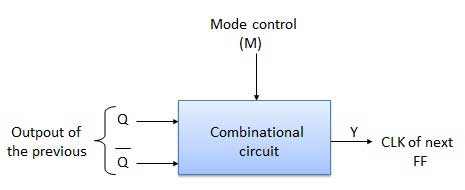### Truth Table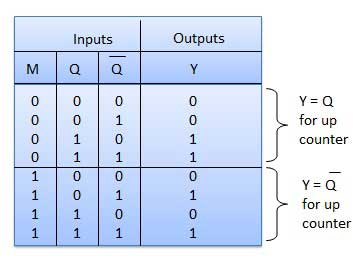### Operation

S.N. Condition Operation
1 Case 1 − With M = 0 (Up counting mode)

If M = 0 and M bar = 1, then the AND gates 1 and 3 in fig. will be enabled whereas the AND gates 2 and 4 will be disabled.

Hence QA gets connected to the clock input of FF-B and QB gets connected to the clock input of FF-C.

These connections are same as those for the normal up counter. Thus with M = 0 the circuit work as an up counter.

2 Case 2: With M = 1 (Down counting mode)

If M = 1, then AND gates 2 and 4 in fig. are enabled whereas the AND gates 1 and 3 are disabled.

Hence QA bar gets connected to the clock input of FF-B and QB bar gets connected to the clock input of FF-C.

These connections will produce a down counter. Thus with M = 1 the circuit works as a down counter.

## Modulus Counter (MOD-N Counter)

The 2-bit ripple counter is called as MOD-4 counter and 3-bit ripple counter is called as MOD-8 counter. So in general, an n-bit ripple counter is called as modulo-N counter. Where, MOD number = 2n.

### Type of modulus

• 2-bit up or down (MOD-4)
• 3-bit up or down (MOD-8)
• 4-bit up or down (MOD-16)

## Application of counters

• Frequency counters
• Digital clock
• Time measurement
• A to D converter
• Frequency divider circuits
• Digital triangular wave generator.